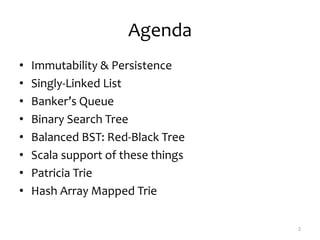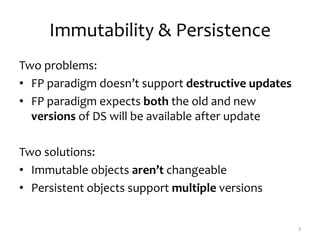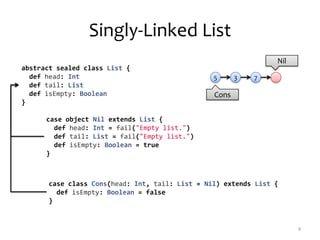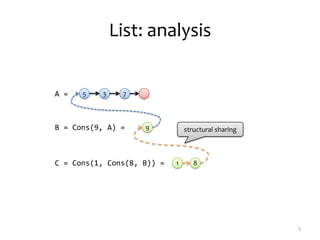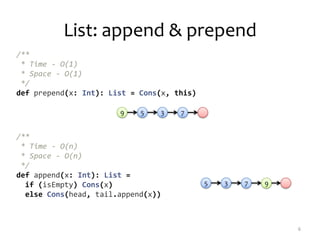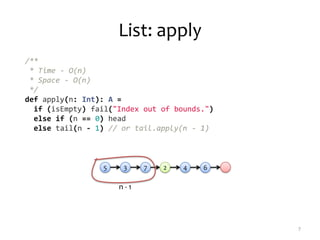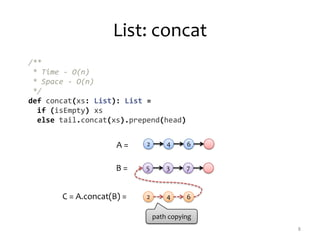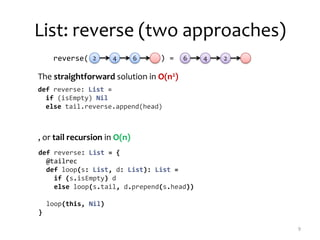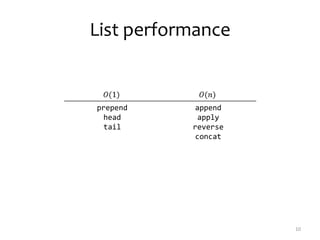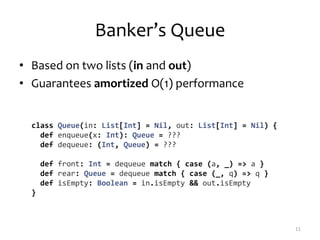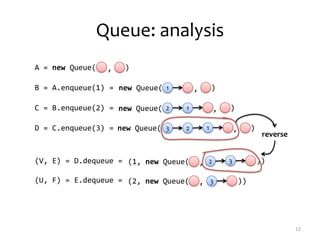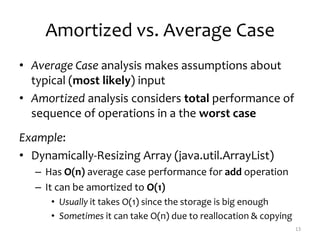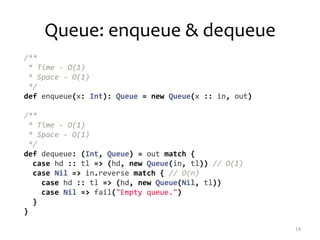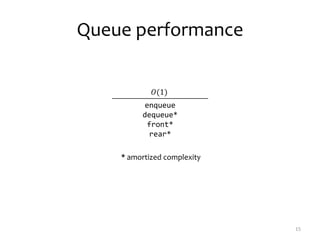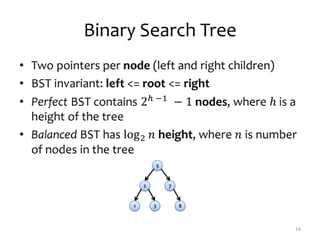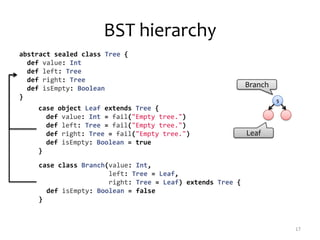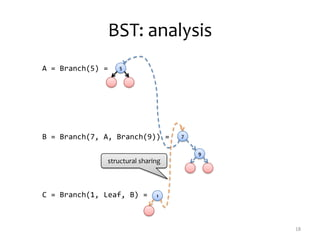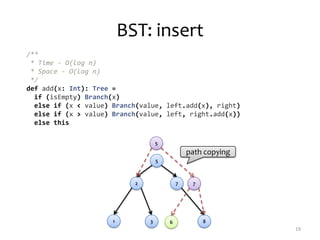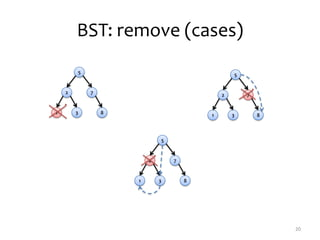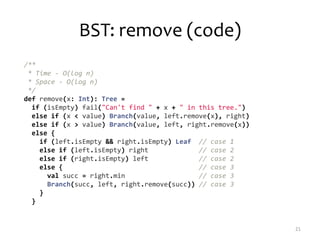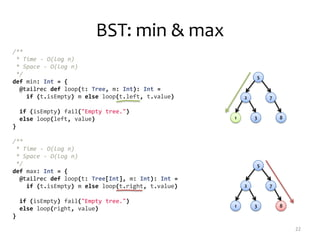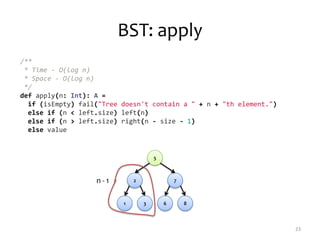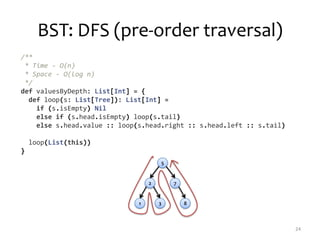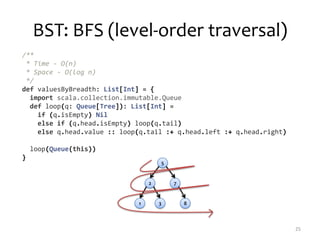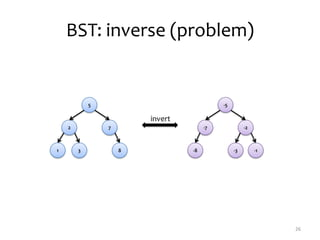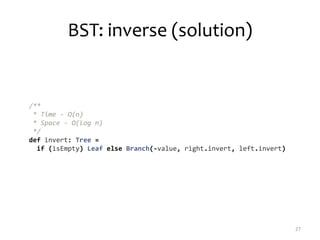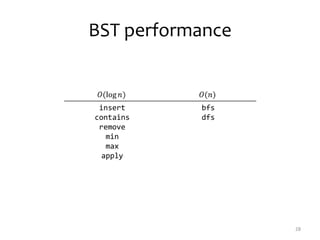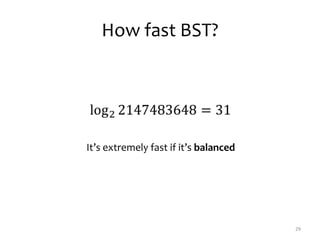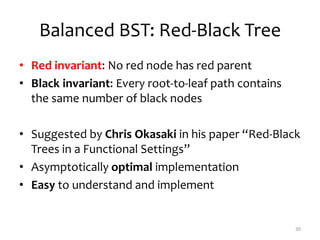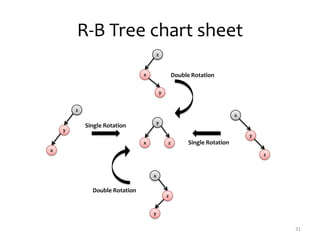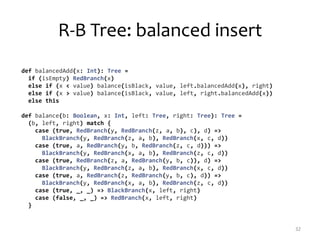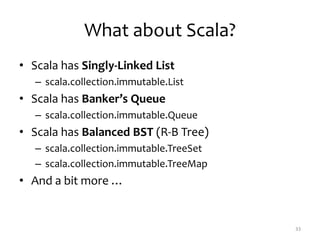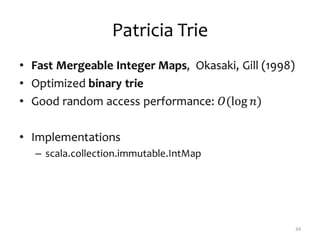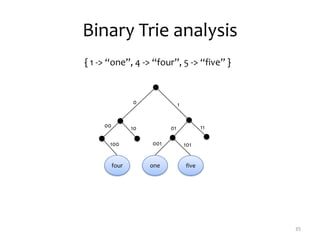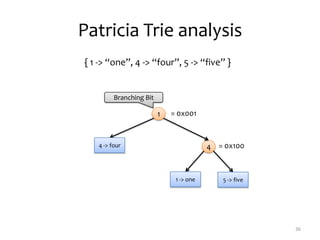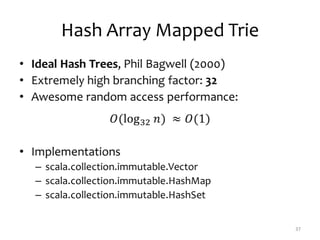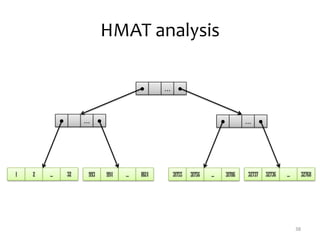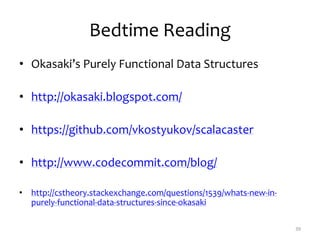1 of 40

### Purely Functional Data Structures in Scala

1. Purely Functional Data Structures in Scala Vladimir Kostyukov http://vkostyukov.ru
2. Agenda • Immutability & Persistence • Singly-Linked List • Banker’s Queue • Binary Search Tree • Balanced BST: Red-Black Tree • Scala support of these things • Patricia Trie • Hash Array Mapped Trie 2
3. Immutability & Persistence Two problems: • FP paradigm doesn’t support destructive updates • FP paradigm expects both the old and new versions of DS will be available after update Two solutions: • Immutable objects aren’t changeable • Persistent objects support multiple versions 3
4. Singly-Linked List 4 35 7 Cons Nil abstract sealed class List { def head: Int def tail: List def isEmpty: Boolean } case object Nil extends List { def head: Int = fail("Empty list.") def tail: List = fail("Empty list.") def isEmpty: Boolean = true } case class Cons(head: Int, tail: List = Nil) extends List { def isEmpty: Boolean = false }
5. List: analysis 5 35 7A = B = Cons(9, A) = 9 C = Cons(1, Cons(8, B)) = 1 8 structural sharing
6. /** * Time - O(1) * Space - O(1) */ def prepend(x: Int): List = Cons(x, this) /** * Time - O(n) * Space - O(n) */ def append(x: Int): List = if (isEmpty) Cons(x) else Cons(head, tail.append(x)) List: append & prepend 6 35 79 35 7 9
7. List: apply 7 35 7 42 6 n - 1 /** * Time - O(n) * Space - O(n) */ def apply(n: Int): A = if (isEmpty) fail("Index out of bounds.") else if (n == 0) head else tail(n - 1) // or tail.apply(n - 1)
8. List: concat 8 path copying A = 42 6 B = 35 7 C = A.concat(B) = 42 6 /** * Time - O(n) * Space - O(n) */ def concat(xs: List): List = if (isEmpty) xs else tail.concat(xs).prepend(head)
9. List: reverse (two approaches) 9 42 6 46 2reverse( ) = def reverse: List = if (isEmpty) Nil else tail.reverse.append(head) , or tail recursion in O(n) The straightforward solution in O(n2) def reverse: List = { @tailrec def loop(s: List, d: List): List = if (s.isEmpty) d else loop(s.tail, d.prepend(s.head)) loop(this, Nil) }
10. List performance 10 prepend head tail append apply reverse concat
11. Banker’s Queue • Based on two lists (in and out) • Guarantees amortized O(1) performance 11 class Queue(in: List[Int] = Nil, out: List[Int] = Nil) { def enqueue(x: Int): Queue = ??? def dequeue: (Int, Queue) = ??? def front: Int = dequeue match { case (a, _) => a } def rear: Queue = dequeue match { case (_, q) => q } def isEmpty: Boolean = in.isEmpty && out.isEmpty }
12. Queue: analysis 12 A = new Queue( , ) B = A.enqueue(1) = 1 , ) C = B.enqueue(2) = 12 , ) D = C.enqueue(3) = 23 1 , ) (V, E) = D.dequeue = , ))2 3 (U, F) = E.dequeue = , ))3 reverse new Queue( new Queue( new Queue( (1, new Queue( (2, new Queue(
13. Amortized vs. Average Case • Average Case analysis makes assumptions about typical (most likely) input • Amortized analysis considers total performance of sequence of operations in a the worst case Example: • Dynamically-Resizing Array (java.util.ArrayList) – Has O(n) average case performance for add operation – It can be amortized to O(1) • Usually it takes O(1) since the storage is big enough • Sometimes it can take O(n) due to reallocation & copying 13
14. Queue: enqueue & dequeue 14 /** * Time - O(1) * Space - O(1) */ def enqueue(x: Int): Queue = new Queue(x :: in, out) /** * Time - O(1) * Space - O(1) */ def dequeue: (Int, Queue) = out match { case hd :: tl => (hd, new Queue(in, tl)) // O(1) case Nil => in.reverse match { // O(n) case hd :: tl => (hd, new Queue(Nil, tl)) case Nil => fail("Empty queue.") } }
15. Queue performance 15 enqueue dequeue* front* rear* * amortized complexity
16. Binary Search Tree 16 5 2 7 1 3 8
17. BST hierarchy 17 abstract sealed class Tree { def value: Int def left: Tree def right: Tree def isEmpty: Boolean } case object Leaf extends Tree { def value: Int = fail("Empty tree.") def left: Tree = fail("Empty tree.") def right: Tree = fail("Empty tree.") def isEmpty: Boolean = true } case class Branch(value: Int, left: Tree = Leaf, right: Tree = Leaf) extends Tree { def isEmpty: Boolean = false } 5 Branch Leaf
18. BST: analysis 18 A = Branch(5) = 5 B = Branch(7, A, Branch(9)) = 7 9 C = Branch(1, Leaf, B) = 1 structural sharing
19. BST: insert 19 5 2 7 1 3 86 path copying 7 5 /** * Time - O(log n) * Space - O(log n) */ def add(x: Int): Tree = if (isEmpty) Branch(x) else if (x < value) Branch(value, left.add(x), right) else if (x > value) Branch(value, left, right.add(x)) else this
20. BST: remove (cases) 20 5 2 7 1 3 8 5 2 7 1 3 8 5 2 7 1 3 8
21. BST: remove (code) 21 /** * Time - O(log n) * Space - O(log n) */ def remove(x: Int): Tree = if (isEmpty) fail("Can't find " + x + " in this tree.") else if (x < value) Branch(value, left.remove(x), right) else if (x > value) Branch(value, left, right.remove(x)) else { if (left.isEmpty && right.isEmpty) Leaf // case 1 else if (left.isEmpty) right // case 2 else if (right.isEmpty) left // case 2 else { // case 3 val succ = right.min // case 3 Branch(succ, left, right.remove(succ)) // case 3 } }
22. /** * Time - O(log n) * Space - O(log n) */ def min: Int = { @tailrec def loop(t: Tree, m: Int): Int = if (t.isEmpty) m else loop(t.left, t.value) if (isEmpty) fail("Empty tree.") else loop(left, value) } /** * Time - O(log n) * Space - O(log n) */ def max: Int = { @tailrec def loop(t: Tree[Int], m: Int): Int = if (t.isEmpty) m else loop(t.right, t.value) if (isEmpty) fail("Empty tree.") else loop(right, value) } BST: min & max 22 5 2 7 1 3 8 5 2 7 1 3 8
23. BST: apply 23 5 2 7 1 3 6 8 n - 1 /** * Time - O(log n) * Space - O(log n) */ def apply(n: Int): A = if (isEmpty) fail("Tree doesn't contain a " + n + "th element.") else if (n < left.size) left(n) else if (n > left.size) right(n - size - 1) else value
24. BST: DFS (pre-order traversal) 24 /** * Time - O(n) * Space - O(log n) */ def valuesByDepth: List[Int] = { def loop(s: List[Tree]): List[Int] = if (s.isEmpty) Nil else if (s.head.isEmpty) loop(s.tail) else s.head.value :: loop(s.head.right :: s.head.left :: s.tail) loop(List(this)) } 5 2 7 1 3 8
25. BST: BFS (level-order traversal) 25 /** * Time - O(n) * Space - O(log n) */ def valuesByBreadth: List[Int] = { import scala.collection.immutable.Queue def loop(q: Queue[Tree]): List[Int] = if (q.isEmpty) Nil else if (q.head.isEmpty) loop(q.tail) else q.head.value :: loop(q.tail :+ q.head.left :+ q.head.right) loop(Queue(this)) } 5 2 7 1 3 8
26. BST: inverse (problem) 26 -5 -7 -2 -8 -3 -1 5 2 7 1 3 8 invert
27. BST: inverse (solution) 27 /** * Time - O(n) * Space - O(log n) */ def invert: Tree = if (isEmpty) Leaf else Branch(-value, right.invert, left.invert)
28. BST performance 28 insert contains remove min max apply bfs dfs
29. How fast BST? 29 It’s extremely fast if it’s balanced
30. Balanced BST: Red-Black Tree • Red invariant: No red node has red parent • Black invariant: Every root-to-leaf path contains the same number of black nodes • Suggested by Chris Okasaki in his paper “Red-Black Trees in a Functional Settings” • Asymptotically optimal implementation • Easy to understand and implement 30
31. R-B Tree chart sheet 31 z y x x y z z y x z x y x z y Double Rotation Double Rotation Single Rotation Single Rotation
32. R-B Tree: balanced insert 32 def balancedAdd(x: Int): Tree = if (isEmpty) RedBranch(x) else if (x < value) balance(isBlack, value, left.balancedAdd(x), right) else if (x > value) balance(isBlack, value, left, right.balancedAdd(x)) else this def balance(b: Boolean, x: Int, left: Tree, right: Tree): Tree = (b, left, right) match { case (true, RedBranch(y, RedBranch(z, a, b), c), d) => BlackBranch(y, RedBranch(z, a, b), RedBranch(x, c, d)) case (true, a, RedBranch(y, b, RedBranch(z, c, d))) => BlackBranch(y, RedBranch(x, a, b), RedBranch(z, c, d)) case (true, RedBranch(z, a, RedBranch(y, b, c)), d) => BlackBranch(y, RedBranch(z, a, b), RedBranch(x, c, d)) case (true, a, RedBranch(z, RedBranch(y, b, c), d)) => BlackBranch(y, RedBranch(x, a, b), RedBranch(z, c, d)) case (true, _, _) => BlackBranch(x, left, right) case (false, _, _) => RedBranch(x, left, right) }
33. What about Scala? • Scala has Singly-Linked List – scala.collection.immutable.List • Scala has Banker’s Queue – scala.collection.immutable.Queue • Scala has Balanced BST (R-B Tree) – scala.collection.immutable.TreeSet – scala.collection.immutable.TreeMap • And a bit more … 33
34. Patricia Trie 34
35. Binary Trie analysis 35 { 1 -> “one”, 4 -> “four”, 5 -> “five” } 0 1 00 10 1101 100 four 001 101 one five
36. Patricia Trie analysis 36 { 1 -> “one”, 4 -> “four”, 5 -> “five” } 1 44 -> four 1 -> one 5 -> five Branching Bit = 0x001 = 0x100
37. Hash Array Mapped Trie 37
38. HMAT analysis 38 ● ... ● ● ... ● ● ... ● 1 2 ... 32 993 994 ... 1024 31755 31756 ... 31786 32737 32736 ... 32768
39. Bedtime Reading • Okasaki’s Purely Functional Data Structures • http://okasaki.blogspot.com/ • https://github.com/vkostyukov/scalacaster • http://www.codecommit.com/blog/ • http://cstheory.stackexchange.com/questions/1539/whats-new-in- purely-functional-data-structures-since-okasaki 39
40. 40 vkostyukov vkostyukov